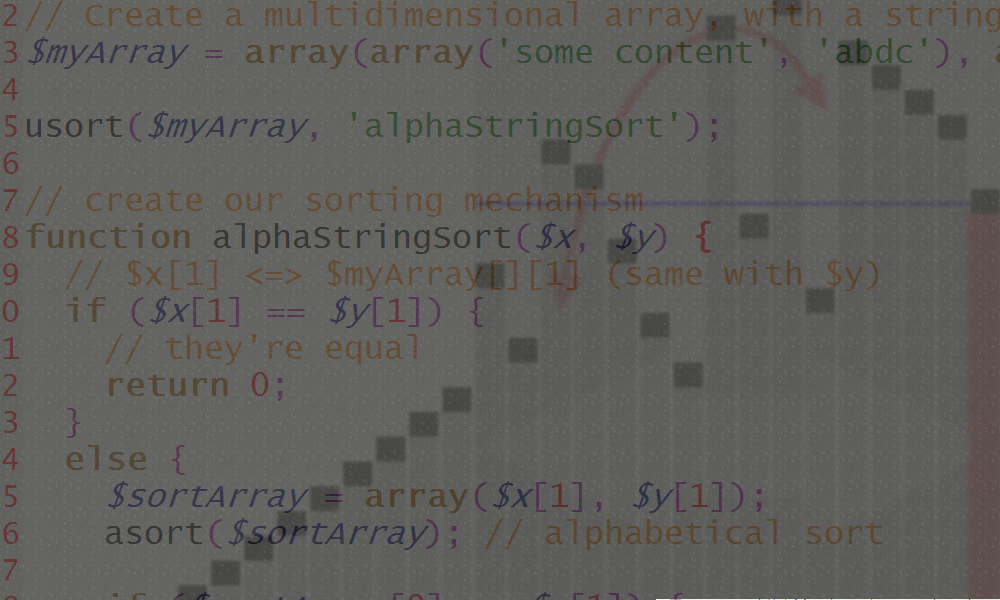# PHP sort arrays by property

2010-11-11If you look at PHP's official comparison of array sorting functions, you'll see that there are several sort functions to sort an array based upon the array member values. However, they don't give you the opportunity to sort multidimensional arrays. At least not without doing some complex tweaking of the functions.

I've found a way that gives you complete control over your sorting mechanism and allows you to easily sort multidimensional arrays. The technique is similar to the way you do it in Java. Even the predefined sorting functions in PHP is using the same technique, but hides it from you.

Let's write our very own customized sort mechanism; we'll have to create a new function and make it look something like this:

``````// Create a multidimensional array, with two random selected
// integers as values
\$myArray = array(array(345, 569), array(123, 456));

// sort the array with our mechanism, second parameter is the name of
// our custom sorting mechanism
usort(\$myArray, 'compareTo');

// create our sorting mechanism
function compareTo(\$x, \$y) {
// \$x <=> \$myArray[] (same with \$y)

if (\$x == \$y) {
// they're equal
return 0;
}
else if (\$x < \$y) {
// \$y is greater
return 1;
} else {
// \$x is greater
return -1;
}
}``````

The multidimensional array (\$myArray) and the usort() method are obvious. But what about the sort function, how does it work?

usort() is using your custom sort function (compareTo()) to compare two objects of your array and sort your array based upon the result. In this case, usort() runs compareTo() like this: compareTo(\$myArray, \$myArray);. Our custom compareTo() is using the first value of each parameter array (\$myArray ><== \$myArray) to return either 0, 1 or -1.

If you want to sort the array based upon the second value instead, just replace \$x and \$y in compareTo() with \$x and \$y. In that way, you can compare any field in any multidimensional array as you want. It even support strings (\$x['myString'])!

The remaining thing to cover is the return value (-1, 0 or 1). 0 means that the objects are equal (the same), 1 that \$x is larger than \$y and -1 that \$y is larger than \$x. The compareTo() in the example above sorts the array descending (largest number at top). Just swap the 1 and -1 return result if you want it to be the opposite (ascending). Another way to do it is to replace the < with an >.

You should now understand how it works and be able to modify it so you can sort anything however you want. This also works for one dimensional arrays. The last thing I should cover is sorting when the values are strings. Whether you sort it alphabetically, based upon string length or something entirely else is up to you. In this example, we're doing it the most common way (alphabetically):

``````// Create a multidimensional array, with a string as the sorting value
\$myArray = array(array('some content', 'abdc'), array('other content', 'abc'));

usort(\$myArray, 'alphaStringSort');

// create our sorting mechanism
function alphaStringSort(\$x, \$y) {
// \$x <=> \$myArray[] (same with \$y)
if (\$x == \$y) {
// they're equal
return 0;
}
else {
\$sortArray = array(\$x, \$y);
asort(\$sortArray); // alphabetical sort

if (\$sortArray == \$x) {
return 1; // \$x is greater (in this case; 'abc')
} else {
return -1; // \$y is greater
}
}
}``````